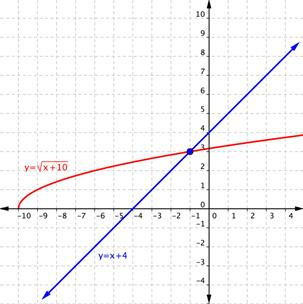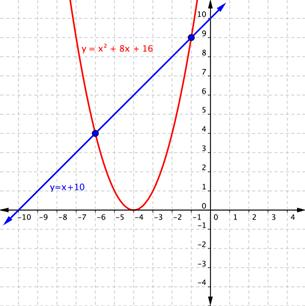### Learning Objectives

• (9.5.1) – Solving Radical Equations
• Identify a radical equation with no solutions, or extraneous solutions
• (9.5.2) – Applications with radical equations
• Kinetic Energy
• Volume
• Free-fall

# (9.5.1) – Solving Radical Equations

Now that you understand how to combine radical terms and expressions using algebraic operations, you can solve equations that contain radicals. Soon, we will be able to help Joan (and Anne) figure out how tall the ladder needs to be!  In this lesson you will use the addition and multiplication properties of equality as well as the rules for exponents.

An equation that contains a radical expression is called a radical equation. Solving radical equations requires applying the rules of exponents and following some basic algebraic principles. In some cases, it also requires looking out for errors generated by raising unknown quantities to an even power.

A basic strategy for solving radical equations is to isolate the radical term first, and then raise both sides of the equation to a power to remove the radical. (The reason for using powers will become clear in a moment.) This is the same type of strategy you used to solve other, non-radical equations—rearrange the expression to isolate the variable you want to know, and then solve the resulting equation.

There are two key ideas that you will be using to solve radical equations. The first is that if $a=b$, then ${{a}^{2}}={{b}^{2}}$. (This property allows you to square both sides of an equation and remain certain that the two sides are still equal.) The second is that if the square root of any nonnegative number $x$ is squared, then you get $x$: ${{\left( \sqrt{x} \right)}^{2}}=x$. (This property allows you to “remove” the radicals from your equations.)

Let’s start with a radical equation that you can solve in a few steps:$\sqrt{x}-3=5$.

### Example

Solve. $\sqrt{x}-3=5$

To check your solution, you can substitute 64 in for $x$ in the original equation. Does $\sqrt{64}-3=5$? Yes—the square root of 64 is 8, and $8−3=5$.

Notice how you combined like terms and then squared both sides of the equation in this problem. This is a standard method for removing a radical from an equation. It is important to isolate a radical on one side of the equation and simplify as much as possible before squaring. The fewer terms there are before squaring, the fewer additional terms will be generated by the process of squaring.

In the example above, only the variable $x$ was underneath the radical. Sometimes you will need to solve an equation that contains multiple terms underneath a radical. Follow the same steps to solve these, but pay attention to a critical point—square both sides of an equation, not individual terms. Watch how the next two problems are solved.

### Example

Solve. $\sqrt{x+8}=3$

In the following video example you will see two more examples that are similar to the ones above.

### Example

Solve. $1+\sqrt{2x+3}=6$

In the next example, we present a radical equation with a radical of index 3:

### ExAMPLE

Solve. $\sqrt{5x+1}+8+4$

1. Isolate the radical expression (index $n$) to one side.
2. Raise both sides of the equation to the $n^{th}$ power : If $x=y$ then $x^{n}=y^{n}$.
3. Once the radical is removed, solve for the unknown. (If necessary, repeat first two steps, until all radicals are removed.)

### Identify a radical equation with no solutions or extraneous solutions

Following rules is important, but so is paying attention to the math in front of you—especially when solving radical equations. Take a look at this next problem that demonstrates a potential pitfall of squaring both sides to remove the radical.

### Example

Solve. $\sqrt{a-5}=-2$

Look at that—the answer $a=9$ does not produce a true statement when substituted back into the original equation. What happened?

Check the original problem: $\sqrt{a-5}=-2$. Notice that the radical is set equal to $−2$, and recall that the principal square root of a number can only be positive. This means that no value for a will result in a radical expression whose positive square root is $−2$! You might have noticed that right away and concluded that there were no solutions for a. But why did the process of squaring create an answer, $a=9$, that proved to be incorrect?

The answer lies in the process of squaring itself. When you raise a number to an even power—whether it is the second, fourth, or 50th power—you can introduce a false solution because the result of an even power is always a positive number. Think about it: $3^{2}$ and $\left(−3\right)^{2}$ are both 9, and $2^{4}$ and $\left(−2\right)^{4}$ are both 16. So when you squared $-2$ and got $4$ in this problem, you artificially turned the quantity positive. This is why you were still able to find a value for $a$—you solved the problem as if you were solving$\sqrt{a-5}=2$! (The correct solution to $\sqrt{a-5}=-2$ is actually “no solution.”)

Incorrect values of the variable, such as those that are introduced as a result of the squaring process are called extraneous solutions. Extraneous solutions may look like the real solution, but you can identify them because they will not create a true statement when substituted back into the original equation. This is one of the reasons why checking your work is so important—if you do not check your answers by substituting them back into the original equation, you may be introducing extraneous solutions into the problem.

Have a look at the following problem. Notice how the original problem is $x+4=\sqrt{x+10}$, but after both sides are squared, it becomes ${{x}^{2}}+8x+16=x+10$. Squaring both sides may have introduced an extraneous solution.

In the following video we present more examples of solving radical equations by isolating a radical term on one side.

### Example

Solve. $x+4=\sqrt{x+10}$

It may be difficult to understand why extraneous solutions exist at all. Thinking about extraneous solutions by graphing the equation may help you make sense of what is going on.

You can graph $x+4=\sqrt{x+10}$ on a coordinate plane by breaking it into a system of two equations: $y=x+4$ and $y=\sqrt{x+10}$. The graph is shown below. Notice how the two graphs intersect at one point, when the value of x is $−1$. This is the value of x that satisfies both equations, so it is the solution to the system.Now, following the work we did in the example problem, let’s square both of the expressions to remove the variable from the radical. Instead of solving the equation $x+4=\sqrt{x+10}$  we are now solving the equation $\left(x+4\right)^{2}=\left(\sqrt{x+10}\right)^{2}$ , or $x^{2}+8x+16=x+10$. The graphs of $y=x^{2}+8x+16$ and $y=x+10$ are plotted below. Notice how the two graphs intersect at two points, when the values of x are $−1$ and $−6$.Although $x=−1$ is shown as a solution in both graphs, squaring both sides of the equation had the effect of adding an extraneous solution, $x=−6$. Again, this is why it is so important to check your answers when solving radical equations!

### Example

Solve. $4+\sqrt{x+2}=x$

In the next video we show another example of radical equations that have extraneous solutions. Always remember to check your answers for radical equations.

In our last example we will use a difference of squares to solve a radical equation with two radicals. You will see that we have to isolate and eliminate one radical first, then tackle isolating and eliminating the other. We will also use factoring techniques for trinomials and the zero product principle to solve this equation.

### Example

Solve $\sqrt{2x+3}+\sqrt{x - 2}=4$.

In our last video we show the solution for another radical equation that has square roots on both sides of the equation. Pay special attention to how you square the side that has the sum of a radical term and a constant.

# (9.5.2) – Applications with Radical Equations

Kinetic EnergyOne way to measure the amount of energy that a moving object (such as a car or roller coaster) possesses is by finding its Kinetic Energy. The Kinetic Energy ($E_{k}$, measured in Joules) of an object depends on the object’s mass ($m$), measured in kg) and velocity ($v$), measured in meters per second), and can be written as $v=\sqrt{\frac{2{{E}_{k}}}{m}}$.

### Example

What is the Kinetic Energy of an object with a mass of 1,000 kilograms that is traveling at 30 meters per second?

Here is another example of finding the kinetic energy of an object in motion.

### Volume

Harvester ants found in the southwest of the U.S. create a vast interlocking network of tunnels for their nests. As a result of all this excavation, a very common above-ground hallmark of a harvester ant nest is a conical mound of small gravel or sandThe radius of  a cone whose height is is equal to twice it’s radius is given as: $r=\sqrt{\frac{3V}{2\pi }}$.

### Example

A mound of gravel is in the shape of a cone with the height equal to twice the radius. Calculate the volume of such a mound of gravel whose radius is 3.63 ft. Use $\pi =3.14$.

Here is another example of finding Volume given the radius of a cone.

## Free-FallWhen you drop an object from a height, the only force acting on it is gravity (and some air friction) and it is said to be in free-fall. We can use math to describe the height of an object in free fall after a given time because we know how to quantify the force of the earth pulling on us – the force of gravity.

An object dropped from a height of 600 feet has a height, $h$, in feet after $t$ seconds have elapsed, such that $h=600 - 16{t}^{2}$. In our next example we will find the time at which the object is at a given height by first solving for $t$.

### Example

Find the time is takes to reach a height of 400 feet by first finding an expression for $t$.

Radical equations play a significant role in science, engineering, and even music. Sometimes you may need to use what you know about radical equations to solve for different variables in these types of problems.

1. Taber, Stephen Welton. The World of the Harvester Ants. College Station: Texas A & M University Press, 1998.